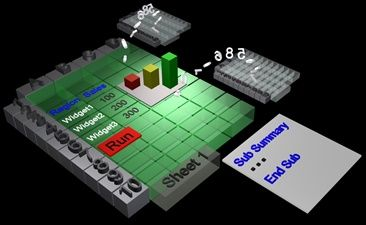VBA (Visual Basic for Applications) is the programming language of Excel and other Office programs.

1. Create a Macro: With Excel VBA you can automate tasks in Excel by writing so called macros. In this chapter, learn how to create a simple macro.

2. MsgBox: The MsgBox is a dialog box in Excel VBA you can use to inform the users of your program.

4. Range Object: The Range object, which is the representation of a cell (or cells) on your worksheet, is the most important object of Excel VBA.

5. Variables: This chapter teaches you how to declare, initialize and display a variable in Excel VBA.

6. If Then Statement: Use the If Then statement in Excel VBA to execute code lines if a specific condition is met.

7. Loop: Looping is one of the most powerful programming techniques. A loop in Excel VBA enables you to loop through a range of cells with just a few codes lines.

8. Macro Errors: This chapter teaches you how to deal with macro errors in Excel.

9. String Manipulation: In this chapter, you'll find the most important functions to manipulate strings in Excel VBA.

10. Date and Time: Learn how to work with dates and times in Excel VBA.

11. Events: Events are actions performed by users which trigger Excel VBA to execute code.

12. Array: An array is a group of variables. In Excel VBA, you can refer to a specific variable (element) of an array by using the array name and the index number.

13. Function and Sub: In Excel VBA, a function can return a value while a sub cannot.

14. Application Object: The mother of all objects is Excel itself. We call it the Application object. The application object gives access to a lot of Excel related options.

15. ActiveX Controls: Learn how to create ActiveX controls such as command buttons, text boxes, list boxes etc.

16. Userform: This chapter teaches you how to create an Excel VBA Userform.